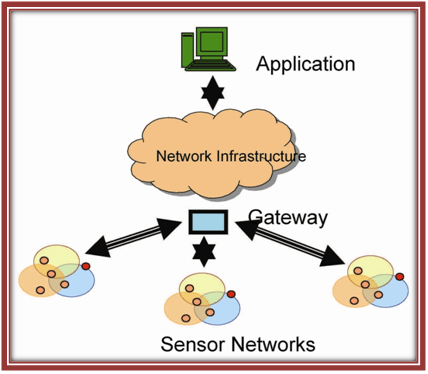Ns3 Projects for B.E/B.Tech M.E/M.Tech PhD Scholars.  Phone-Number:9790238391   E-mail: ns3simulation@gmail.com

# NS3 for WSN

Wireless sensor network is a collection of nodes organized into a cooperative network.Each node consists of processing capability, may contain multiple types of memory, have a RF transceiver, have a power source, and accommodate various sensors and actuators.Ns3 WSN system incorporates a gateway that provides wireless connectivity back to the wired world and distributed nodes. The wireless protocol you select depends on your application requirements.

Applications of WSN ( Wireless Sensor Network):

• Asset tracking.
• Periodic measurements.
• Environmental and monitoring of air, water and soil.
• Event detection.
• Process monitoring.
• Industrial machine monitoring.
• Structural monitoring for buildings and bridges.

Components of WSN node:

• Sensor interface.
• Analog circuit.
• Battery.
• Microcontroller etc.

Need for introducing WSN:

• Production cost.
• Fault tolerance.
• Security.
• Scalability.
• Real time.

Architecture of WSN:Sample code for WSN:

```
{
return degrees * M_PI / 180.0;
}
{
return radians * 180.0 / M_PI;
}
std::ostream& operator<< (std::ostream& os, const Angles& a)
{
os << "(" << a.phi << ", " << a.theta << ")";
return os;
}
std::istream &operator >> (std::istream &is, Angles &a)
{
char c;
is >> a.phi >> c >> a.theta;
if (c != ':')
{
is.setstate (std::ios_base::failbit);
}
return is;
}
Angles::Angles ()
: phi (0),
theta (0)
{
}
Angles::Angles (double p, double t)
: phi (p),
theta (t)
{
}
Angles::Angles (Vector v)
: phi (std::atan2 (v.y, v.x)),
theta (std::acos (v.z / sqrt (v.x*v.x + v.y*v.y + v.z*v.z)))
{
}
Angles::Angles (Vector v, Vector o)
: phi (std::atan2 (v.y - o.y, v.x - o.x)),
theta (std::acos ((v.z - o.z) / CalculateDistance (v, o)))
{
}

```